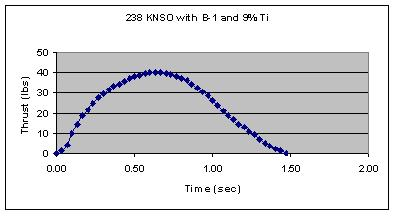Engine Test data is presented on this page using various additives that act either as fuels (i.e. Al and Ti powder), or burn rate modifiers.  These motor tests were run in standard KNO3/sugar formulations based on a 65:35 weight ratio with the sugar amount reduced by the amount of additive present.  All tests were also run using Polystep B-1 viscosity reducer present as well.
Ti powder; <100 mesh, fines from low priced "sweepings", SkylighterThrust Duration = 1.5 sec
Propellant wt.  = 0.284 lb
Throat area  =  0.0248 in2
Max. Thrust = 40.1 lbs
Max. Chamber Pressure = 1078 lbs
Ave thrust = 24.4 lbs  =  108.7 N
Total impulse  =  36.6 lb sec  =  163 N sec
ISP = 129
Motor class  = H109
Al Powder; Chinese salvaged

Results soon to come
Fe (II) Gluconate; 1% burn rate enhancer
238 KNSO B-1 1% FeGluconate
Propellant mass = 121.4 g = 0.267 lbs
Throat area = 0.0372 in2
Thrust duration = 1.41 sec
Max Thrust = 35 lbs
Max Chamber Pressure = 675 psi
Ave Thrust = 23.5 lbs = 105 N
Total Impulse = 147 N sec = 33.1 lb sec
ISP = 124 sec
Motor class = G 105
Fe (II) Gluconate; 5% burn rate enhancer
238 KNSO B-1 and 5% FeGluconate
Thurst Duration = 1.12 sec
Propellant Mass = 123 g = 0.271 lb
Throat area = 0.0372 in2
Max Thrust = 61.5 lbs
Max Chamber Pressure = 1182 psi
Ave. Thrust = 31.7 lbs = 141 N
Total impulse = 158 N sec = 35.5 lb sec
ISP = 131
Motor class = G141